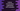# Enumeration in python with examples## Enumeration in python :

Using enumeration can improve the readability of your code. Enumeration is a set of key-value pairs with each key is a symbolic name bound to its value. You can use the symbolic name in your code using enumeration to make it more readable.

Python provides one module called enum to create enumerations. In this post, I will show you how to create enumeration in python and different operations that we can perform.

## Create an enum using class:

In python, enums are created using classes. You need to subclass the Enum class like below :

``````from enum import Enum

class Days(Enum):
SUN = "sun"
MON = 1
TUES = 2
WED = 3

print("{} : {}".format(repr(Days.SUN),Days.SUN.value))
print("{} : {}".format(repr(Days.MON),Days.MON.value))
print("{} : {}".format(repr(Days.TUES),Days.TUES.value))
print("{} : {}".format(repr(Days.WED),Days.WED.value))``````

It will print :

``````<Days.SUN: 'sun'> : sun
: 1
: 2
: 3``````

Here for each key of the enum, one value is assigned. You can have two keys with the same value but keys should be always unique. It will throw one error if you reuse a key.

## Creating enum using functional API :

We can also create enums using functional API like below :

``````from enum import Enum

e = Enum('e','Dog Cat Cow')

print(e.Dog.value)
print(e.Cat.value)
print(e.Cow.value)``````

It will print :

``````1
2
3``````

The first method is preferred.

### Accessing key-value pairs :

Different ways are available to access the key-value pairs of an enumeration. We can access each item by its key or value :

``````from enum import Enum

class Days(Enum):
SUN = "sun"

print('{} : {}'.format(Days.SUN.name, Days.SUN.value))
print('{} : {}'.format(Days["SUN"].name, Days["SUN"].value))
print('{} : {}'.format(Days("sun").name, Days("sun").value))``````

Output :

``````SUN : sun
SUN : sun
SUN : sun``````

### Iterate :

You can iterate through the items of enumeration :

``````from enum import Enum

class Days(Enum):
SUN = "sun"
MON = 1
TUES = 2
WED = 3

for item in Days:
print(item)
print(item.value)``````

Output :

``````Days.SUN
sun
Days.MON
1
Days.TUES
2
Days.WED
3``````

### Using two keys with the same value :

``````from enum import Enum

class Days(Enum):
SUN = "sun"
MON = 1
TUES = 1
WED = 1

for item in Days:
print(item)
print(item.value)``````

It will print :

``````Days.SUN
sun
Days.MON
1``````

It will ignore TUES and WED. But you can print the values of these keys like print(Days.TUES.value) will print 1.

### Use in a dictionary and set :

Each member of the enumeration is hashable. So, we can use them in dictionaries and sets.

``````from enum import Enum

class Days(Enum):
SUN = "sun"
MON = "mon"

my_dict = {}
my_dict[Days.SUN] = 1
my_dict[Days.MON] = 2

print(my_dict)``````

### Output :

``{<Days.SUN: 'sun'>: 1, <Days.MON: 'mon'>: 2}``

### Comparing enum values :

We can also compare enum values :

``````from enum import Enum

class Days(Enum):
SUN = 1
MON = 2

if Days.SUN != Days.MON:
print("{} is not equal to {}".format(Days.SUN.value, Days.MON.value))``````